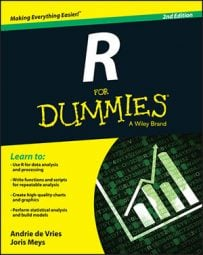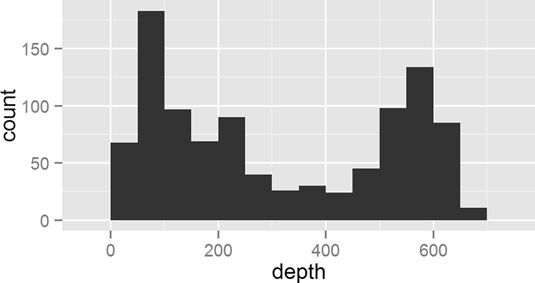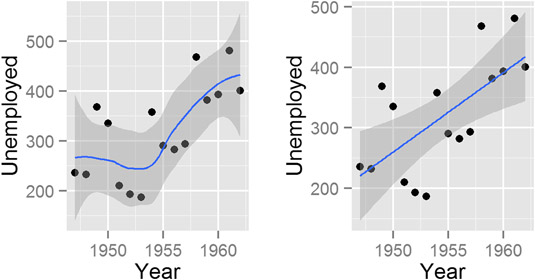##### R For DummiesAfter data, mapping, and geoms, the fourth element of a ggplot2 layer in R describes how the data should be summarized. In ggplot2, you refer to this statistical summary as a stat.

One very convenient feature of ggplot2 is its range of functions to summarize your data in the plot. This means that you often don’t have to pre-summarize your data. For example, the height of bars in a histogram indicates how many observations of something you have in your data. The statistical summary for this is to count the observations. Statisticians refer to this process as binning, and the default stat for geom_bar() is stat_bin().

Analogous to the way that each geom has an associated default stat, each stat also has a default geom.

So, this begs the question: How do you decide whether to use a geom or a stat? In theory it doesn’t matter whether you choose the geom or the stat first. In practice, however, it often is intuitive to start with a type of plot first — in other words, specify a geom. If you then want to add another layer of statistical summary, use a stat.geom_bar()." width="535"/>
Making a histogram with geom_bar().

In this plot, you used the same data to first create a scatterplot with geom_point(), and then you added a smooth line with stat_smooth().

Here some practical examples of using stat functions.

Stat Description Default Geom
stat_bin() Counts the number of observations in bins. geom_bar()
stat_smooth() Creates a smooth line. geom_line()
stat_identity() No summary. Plots data as is. geom_point()
stat_boxplot() Summarizes data for a box-and-whisker plot. geom_boxplot()

## Binning data

You’ve already seen how to use stat_bin() to summarize your data into bins, because this is the default stat of geom_bar(). This means that the following two lines of code produce identical plots:

```> ggplot(quakes, aes(x = depth)) + geom_bar(binwidth = 50)
> ggplot(quakes, aes(x = depth)) + stat_bin(binwidth = 50)```

## Smoothing data

The ggplot2 package also makes it very easy to create regression lines through your data. You use the stat_smooth() function to create this type of line.

The interesting thing about stat_smooth() is that it makes use of local regression by default. R has several functions that can do this, but ggplot2 uses the loess() function for local regression. This means that if you want to create a linear regression model, you have to tell stat_smooth() to use a different smoother function. You do this with the method argument.

To illustrate the use of a smoother, start by creating a scatterplot of unemployment in the longley dataset:

```> p <- ggplot(longley, aes(x = Year, y = Employed)) + geom_point()
> p```

`> p + stat_smooth()`

Your graphic should look like the plot to the left of the image below.

Sometimes, ggplot2 generates messages with extra tips and information. As long as you don’t see warning or error, you can safely ignore these messages. In this case, stat_smooth() tells you that the default smoother is a method called loess (local smoothing). The message also says you can use alternative smoothing methods.

Finally, use stat_smooth() to fit and plot a linear regression model. You do this by adding the argument method=lm:

`> p + stat_smooth(method = "lm")`

Your graphic should now look like the plot to the right.stat_smooth()." width="535"/>

## Doing nothing with identity

Sometimes you don’t want ggplot2 to summarize your data in the plot. This usually happens when your data is already pre-summarized or when each line of your data frame has to be plotted separately. In these cases, you want to tell ggplot2 to do nothing at all, and the stat to do this is stat_identity(). You probably noticed that stat_identity is the default statistic for points and lines.